MINERALS

Density

Density or specific gravity is the ratio of the weight of a substance to the weight of an equal volume of water.

Density = Weight/Volume

# Mineral Density Test Video

 Steps to determine the density of a mineral.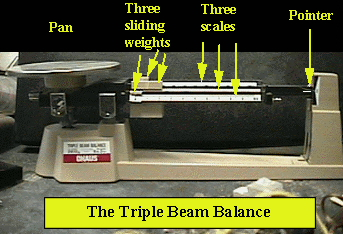1) Use a balance. In this example the balance to be used is a triple beam balance.2) Place the specimen in the weighing pan.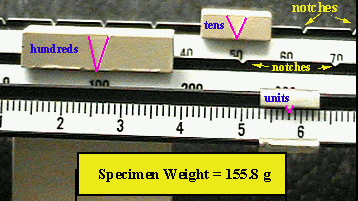3) Record the weight of the specimen, in this case 155.8 grams.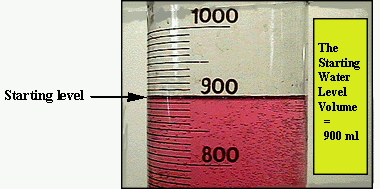4) Record the level in a graduated cylinder before you put the specimen in. In this case 900ml.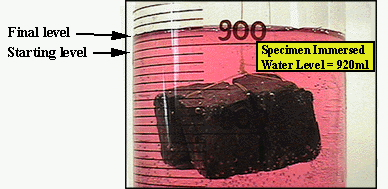5) Record the level after the specimen was placed under water. In this case 920ml. Density = 155.8grams / 20 cc 6) Divide 155.8/20 = 7.79 g/cc. (in this case 20ml = 20cc, because the amount of units displaced are equivalent). So, the density of the minerals is 7.79g/cc.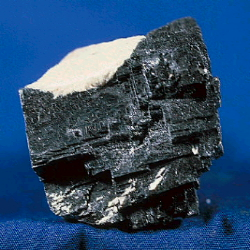7) The closest minerla having this density is galena with a density of 7.60 g/cc. (In order to get a precise density sophisticated equipment with a tolerance of five to seven decimals is used).